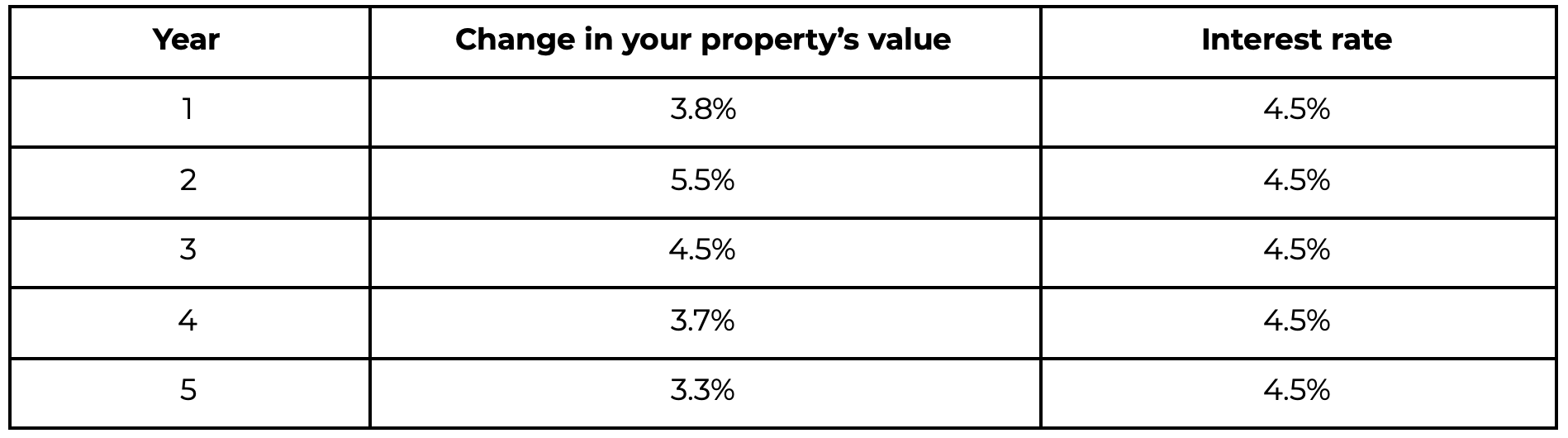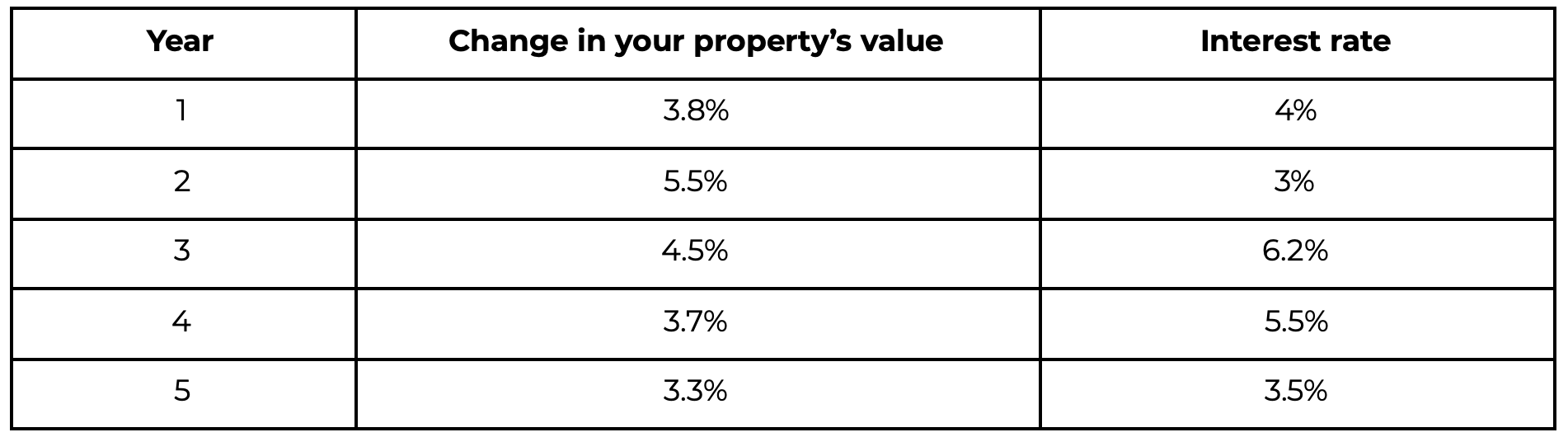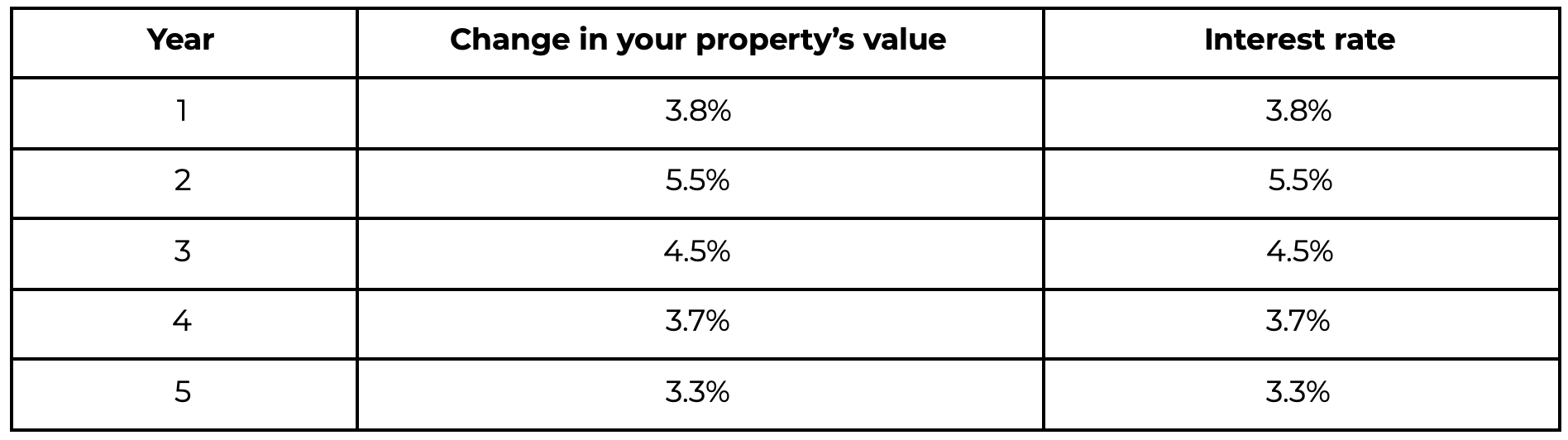# Calculating the cost of a Fraction Mortgage

We often get the question on how the cost of the Fraction Mortgage is calculated. This post lays out exactly how we determine the final cost of the loan.Josh Baker
September 22, 2021
Blog overview

Fixed interest rate mortgages

Variable interest rate mortgages

A Fraction Mortgage

How is the growth of the home’s value determined?

Minimum and maximum interest rates

How much will it cost at the end?

So why Fraction?

Appendix

We often get the question on how the cost of the Fraction Mortgage is calculated. This post is intended to allow us to lay out exactly how we determine the final cost of the loan.

Broadly, there are two main questions we get. The answer to the first question informs the answer to the second question, but it’s helpful to break them up:

1. What will the interest rate of the mortgage be?
2. How much will I owe at the end of the mortgage?

The fundamental difference between a Fraction Mortgage and any other mortgage is how the interest rate is decided. Before we dive into those differences, it’s helpful to discuss the different types of interest rates for traditional products. If you already know how traditional mortgage interest rates work, feel free to skip ahead.

## Fixed interest rate mortgages

These are the simplest to understand, and are the most popular (74% of mortgages in Canada are fixed interest rate mortgages). A fixed interest rate is a single number that doesn’t change during the course of the entire term, which means you will know exactly how much interest you will pay over the course of the mortgage.

Over 5 years, a fixed interest rate could look like this (these are arbitrary numbers — not historical):According to ratehub, the average 5-year fixed interest rate over the last 15 years was 3.22%.

## Variable interest rate mortgages

A variable interest rate mortgage is the second most popular type of mortgage. For reference, 21% of mortgages in Canada are variable interest rate mortgages — the remaining 5% are hybrid mortgages that are a combination of fixed and variable. A variable interest rate can change during the term. This means, unlike a fixed interest rate mortgage, you won’t know exactly how much interest you will pay over the course of the mortgage.

The interest rate for a traditional variable interest rate mortgage is based on the prime rate, which is set by the Bank of Canada. The Bank of Canada decides the prime rate based on various economic factors, including:

• Inflation
• Employment figures
• GDP
• Manufacturing numbers
• Policy and goals of the central bank

Your bank or lender will then usually have their own prime rate, which might be a slight adjustment to the Bank of Canada’s prime rate.

Over 5 years, a variable interest rate could look like this (these are arbitrary numbers, not historical):According to ratehub, the average 5-year variable interest rate over the last 15 years was 2.57%.

## A Fraction Mortgage

A Fraction Mortgage is a variation of a shared appreciation mortgage. Fundamentally, the interest rate is variable, just like a variable interest rate mortgage. The difference between the Fraction Mortgage’s rate and a traditional variable interest rate is how the rate is decided.

Unlike a traditional variable interest rate, which is based on the central bank’s prime rate, the rate of a Fraction Mortgage is based on the growth rate of your specific property’s value.

As you can see from the table below, the interest rate each year is directly based on the growth of the home’s value each year.According to Teranet’s House Price Index, the median property in Canada has increased 6% per year in the last 10 years.

## How is the growth of the home’s value determined?

The chart above assumes we know the growth of the home each year (which we don’t actually know). But for the purposes of calculating the growth rate, all you need to know is the starting value and the end value. Here’s how:

1. When you apply for a Fraction Mortgage, Fraction orders an appraisal from a third party appraiser. The third party appraiser is not affiliated with Fraction (so will give an impartial valuation), and, after doing an in-person (hopefully remote one day!) visit to the property, will provide a value for the home. Let’s call that value V1.
2. At the end of the loan (whether the term is up, you decide to pay Fraction out, or you sell your home), Fraction orders another appraisal, again from a third-party appraiser. They provide a new value for the home, which we will call V2.

We then calculate the average annual growth rate over the time period using the following formula (warning, math incoming):

So as an example, if your home was worth \$500,000 from the first appraisal, and 5 years later it is worth \$600,000, then we would calculate the annual growth rate as the following:

So in this scenario, the Fraction interest rate is 3.713% per year.

(See 1.0 in the appendix for checking this work)

## Minimum and maximum interest rates

The final thing to note with Fraction’s interest rate is that, while it is based on the growth rate of the home, there are minimum and maximum rates.

The minimum rate as of March 2022 is 4.75%. This means that if the average annual growth rate is determined to be less than 4.75%, Fraction’s interest rate will be set at 4.75% per year.

The maximum rate as of March 2022 is 9.75%. This means that if the average annual growth rate is determined to be greater than 9.75%, Fraction’s interest rate will be capped at 9.75% per year.

Why the cap? We figured if we are putting a minimum rate, it’s only fair that we also put a maximum as well. Just seems right.

## How much will it cost at the end?

TLDR: At the start, if your initial Fraction mortgage loan size is 30% of your home’s value it will cost 30% of your home’s value at the end. The exceptions to this rule are if your house grew less than our minimum rate per year or more than our maximum rate per year. See our latest rates here.

There are essentially two dimensions to answering this question with all mortgages:

• What is the interest rate?
• What are the payments?

(There are more factors like compounding periods, whether it is simple or compounding interest, and payment frequency, but let’s keep it simple and ignore all that for now.)

• The interest rate affects how much it costs because it determines how the principal amount of the loan grows over time.
• The payments affect how much principal there is on the loan.

Generally, when you think of a mortgage, most people think of a loan that you would typically use to purchase your first house. Such a loan is likely going to be an amortized mortgage. An amortized loan is one where you make consistent, periodic payments that get applied to both the principal and the interest. At the end of the amortization period, you have fully paid off the loan.

Calculating how much an amortized loan will cost in total (including interest) is actually somewhat complicated, so many helpful online calculators have been made to help people figure out this cost.

The Fraction Mortgage is not an amortized mortgage. There are no monthly payments with a Fraction Mortgage. In fact, you only pay when you are buying Fraction out.

Because of the fact that there are no payments during the term, it is much simpler to calculate the cost of the Fraction Mortgage. You just use the same formula you would use to calculate the value of your home over time: the compound interest formula.

I’m a big fan of Excel/Google Sheets, so here’s the formula to calculate the cost of a Fraction Mortgage over the term. You can just plug in the initial_loan_value and growth_rate variables.

=initial_loan_value * (1 + MIN(MAX(growth_rate, 4.75%), 9.75%))^t

If you want to know what the minimum & maximum potential amount you’d pay over the term, this would be:

Minimum=Initial_loan_value * (1 + 4.75%)^t

Maximum=Initial_loan_value * (1 + 9.75%)^t

For reference, according to Teranet’s House Price Index, the median property in Canada has increased 6% per year in the last 10 years. Note there can be pretty large variance across different areas like Toronto (with 8.35% growth) compared to other areas like Quebec City (2.15% growth).

## So why Fraction?

Fraction is all about flexibility. With no monthly payments, you have increased flexibility to live your life as you want to. You can pay out your existing mortgage or other debts and live payment free.

On average, a homeowner who partners with Fraction ends up spending half as much each month towards paying off existing debt compared to before partnering with Fraction.

Our commitment to financial flexibility is reflected in our terms as well. We have no penalties for buying out Fraction at any time during the term.

While reading through this, if you were paying close attention to the historical numbers provided, you probably noticed that the minimum rate for a Fraction Mortgage is generally higher than the rate for a HELOC or primary mortgage.

The main reason for this is because of our commitment to flexibility. Providing this additional flexibility just costs more. When building Fraction, we looked at what products were available on the market, and we saw that everyone was racing for the lowest rates, but they were ignoring people whose primary concern is flexibility and monthly payments — not rates.

Do we think the Fraction Mortgage is for everyone? Not necessarily. If you can make monthly payments easily and it doesn’t impact your life or your financial health, then a HELOC or traditional primary mortgage with their rate-focused approach might be the right product for you. However, if your primary goal is the flexibility that comes with Fraction — then check us out and apply today!

## Appendix

### 1.0 Annual growth rate calculation‍

If we plug in the growth rate into the compound interest formula, we get:

The math checks out.